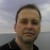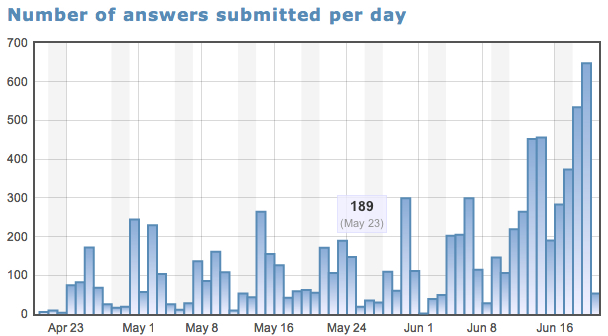# Community posts

## Using PeerWise to assign homeworkby Jan H. Jensen
University of Copenhagen

PeerWise

This past quarter I used a new tool called PeerWise in a physical chemistry course I co-taught. The main idea behind PeerWise, which is a free web-based service developed by Paul Denny at the University of Auckland, is that students write multiple choice questions for each other. I didn't quite have the courage to say "Want homework? Write your own" so instead I used it as a supplement to the normal homework assignments, i.e. I reformulated most of the homework assignments as multiple choice questions and posted them on PeerWise, in addition to the usual homework assignment.

Some information about the course

The course is attended by about 170 chemistry and biochemistry majors and covers thermodynamics and kinetics - more specifically chapters 13-20 in Atkin's Quanta Matter and Change. It's taught in a 9-week quarter and the students take one other course at the same time. There are four other faculty members involved who also pick homework problems associated with their lectures. The students were divided into six teams of roughly 30, and each team has six contact hours with TAs who can help them with the assignments.

1. Feedback to the students. PeerWise allows you to give extensive descriptions (you can even use videos: example 1 and example 2) on how to solve the problem immediately after the student has picked an answer. The quality of the help provided by the TAs tend to vary greatly, and I hoped this would help even things out a bit.
2. Breaking complex problems up into more manageable pieces. A standard question usually asks you to compute x given some conditions. In PeerWise this can be broken up into, for example, a) which of the following equations would you use to compute x? and then b) what is the value x given these conditions. Answering the first question lets people now whether they are on the right track before proceeding.
3. Feedback to me. Students can leave questions or comments for each problem, which often helps me improve the explanation or identify errors in the problem. Students can also rate the quality and difficulty of each question, which is very valuable. I also get all sorts of detailed data including how many students answered the question correctly (more on that below).

Some data

I put 120 questions up on PeerWise and 119 students answered at least one question. From what I understand many students worked in pairs and only one would answer on PeerWise. 39 students answered 100 questions or more. For almost all problems, the majority of students picked the right answer, so they are not just clicking randomly to get the explanation as one could have feared. For example, for the question with the most (86) answers was answered correctly by 57% while the question voted the hardest was answered correctly by 47% of the 76 students who answered it.

While I encouraged students to write their own questions, only 9 questions were written. One of these questions was "Do you find PeerWise helpful?" 41 students answered, 38 said yes. Also several students commented positively on PeerWise with one caveat (see below).Clearly, many students used PeerWise as a study aid before the exam as seen on this figure.

One main problem: errors

The main complaint by the students was the many errors that crept in to the questions, multiple choice answers, and solutions. The multiple choice format exacerbates the problem, since many students will work for hours trying to reproduce one of the answers. This is of course impossible if there is an error and this is very frustrating to the student!

There are several sources of errors.
1. Several errors were introduced by me when I typed in the multiple choice answers (for example, using Joules when I meant kiloJoules) and explanations.
2. Several errors were already in the problems and was copied into PeerWise.
3. Several errors were in the answers provided in the solution manual of the text book and were copied into PeerWise by me. Often the you would simply get a different numerical answer based on the numbers provided.

The error rate was about 1-2 questions per week and all (hopefully) will be fixed next year.

Tips

1. By default the questions are listed in the reverse order they are created (i.e. newest one first), so in cases where the order was important I would write the first question last, e.g. exercise 1b and then exercise 1a.
2. Each question comes with a one line preview, so the first sentence in the question should be the name of the exercise, e.g. Exercise 1a.
3. Each question can also be labelled by subject, so I would use labels like "week 1". With one click one can then display the questions on for a single week only.
4. Many of the comments left by the students said "I punch the solution into my calculator and get a different answer". It's of course hard to know what the problem is, but in these cases I leave a link to the worked out solution on Wolfram|Alpha (example), which is basically on on-line calculator.
5. You add equation through one of several editors (I recommend using the Latex editor) that create and image of the equation, which you can't edit. If you view the page in HTML format there is a Latex code you can copy and paste into the editor and change, rather than typing everything in from scratch.

Changes for next year (When was the last time you learned anything by doing it once?)

Overall PeerWise was a hit, and I will use it next year for this course. I plan to make the following changes:
1. I will add 10-20 math and conceptual training questions every week. Questions one should be able to answer in 1 second: What is e-0? If delta G theta < 1 what can you say about the equilibrium constant? Some of these will show up every week!
2. I will add 1-2 "regular" questions based on topics from previous weeks.
3. I will try to have the explanations include a screenshot of the solution done in Maple. For three reasons: a) it will make me double check the answer in the solution manual, b) it will remove one source of error if I don't have to retype something, and c) it will encourage the students to use Maple (students who use Maple efficiently are done in a fraction of the time compared to others).

Finally, I am racking my brain on how to get more students to write their own questions.